# Add/Subtract Fractions & Mixed Numbers w/ Regrouping

##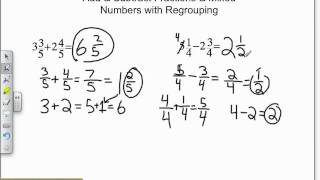By Mathademics

Add/Subtract Fractions & Mixed Numbers w/ Regrouping# Add Subtract Multiply Divide Multi-digit Numbers & Simple Fractions Kids Learning Videos

##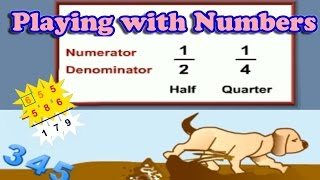By KidsEduc – Kids Educational Games

Add Subtract Multiply and Divide Numbers and Simple Fractions# Adding and Subtracting Complex Numbers

##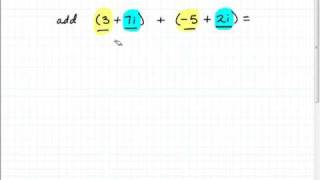By mathonpoint

This video explains how to add or subtract complex numbers. Two example problems are completed in a step-by-step manner.# Adding and subtracting negative fractions

##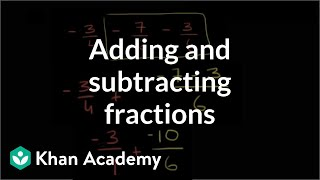By Khan Academy

Add and subtract negative fractions with unlike denominators.##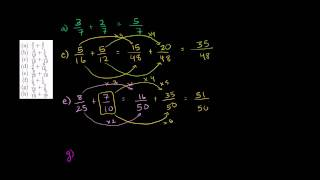By Khan Academy##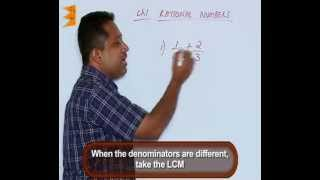By Escholarindia Faridabad##By Khan Academy

Sal defines what it means to add or subtract matrices. He shows a few examples and discusses some important properties of matrix addition and subtraction.# Grade 2 math 11.10a adding and subtracting money (dollars and cents)

##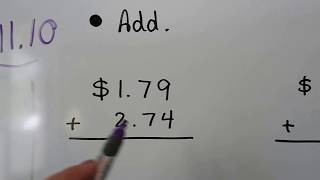By Joann's School

How to add or subtract money in vertical form as the amounts stacked one on top of the other. A two part word problem is included involving addition and subtraction of money.# Add and subtract polynomials with two variables

##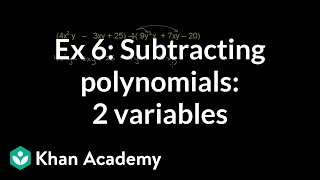By Khan Academy

Sal simplifies (4x^2y - 3xy + 25) - (9y^2x + 7xy - 20).# Add and subtract polynomials with two variables

##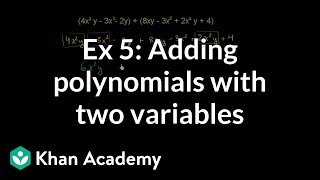By Khan Academy

Sal simplifies (4x^2y - 3x^2 - 2y) + (8xy - 3x^2 + 2x^2y + 4).# Add and subtract polynomials with two variables

##By Khan Academy

Sal subtracts -6x^4-3x^2y^2+5y^4 from 2x^4=8x^2y^2-y^4.# How to Pass the Math FSA (4th) - Add and Subtract

##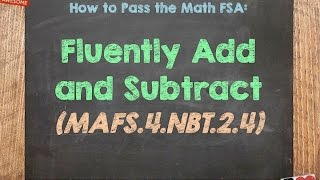By McCarthyAcademy

how to solve 4th Grade FSA Math-style problems. The standard covered in this video is MAFS.4.NBT.2.4# Add and subtract polynomials with one variable

##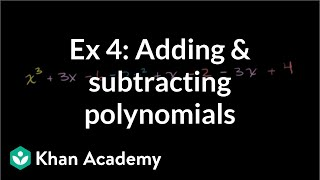By Khan Academy

Sal simplifies (x^3 + 3x - 6) + (-2x^2 + x - 2) - (3x - 4).# Add and subtract polynomials with one variable

##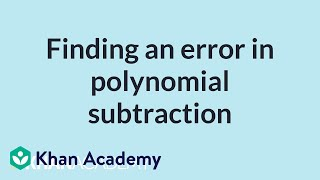By Khan Academy

Sal analyzes a polynomial subtraction process to find the step that has an error.# Add and subtract polynomials with one variable

##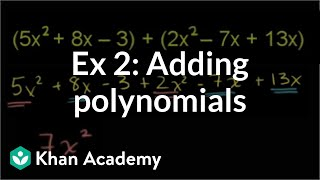By Khan Academy

Sal simplifies (5x^2 + 8x - 3) + (2x^2 - 7x + 13x).# Add and subtract polynomials with one variable

##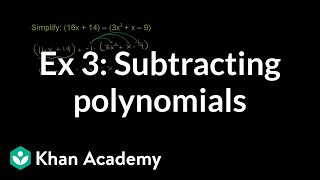By Khan Academy

Sal simplifies (16x+14) - (3x^2 + x - 9).# 7.NS.A.1b / 7.NS.A.1c - Addition and Subtraction of Rational Numbers (Part 1 of 2)

##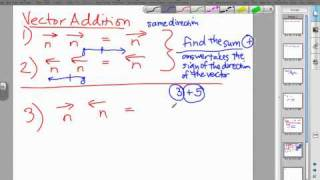By MathwithMrAlmeida

Watch Mr. Almeida fully explain how to add and subtract rational numbers using vectors and the concept of a number line.# One-step equations with addition and subtraction

##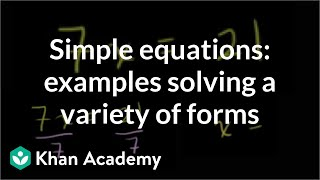By Khan Academy

Some quick examples to practice solving a variety of one step equations. All 4 operations (add, subtract, multiple, divide) are paired with variables.##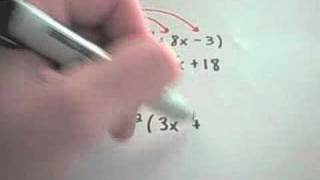By PatrickJMT

In this video, the instructor defines polynomials, then gives some examples of how to add and subtract polynomials. He works through the steps of example problems to explain how to work add and subject polynomials. His explanations are clear and thorough and it would be easy to follow along as he explains.##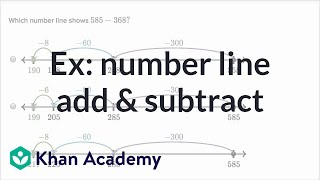By Khan Academy